# Bit Manipulation with C++20

This post concludes my presentation of library features in C++20. Today I am writing about the class` std::source_location` and a few functions for bit manipulation.

##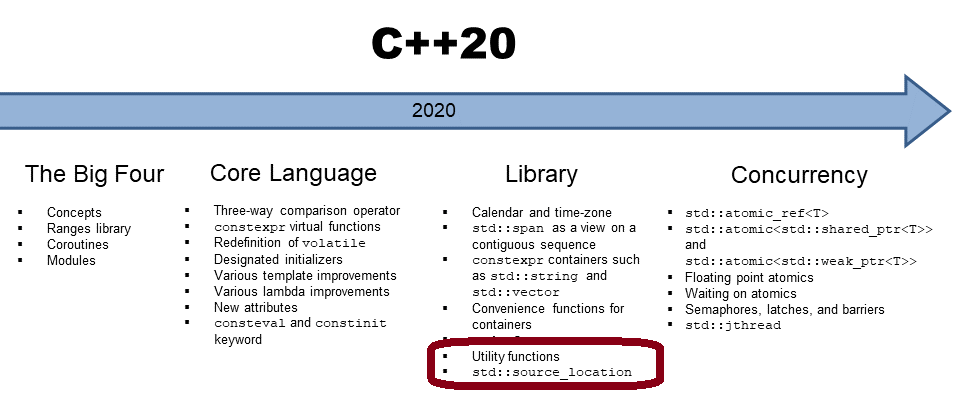`std::source_location`

`std::source_location` represents information about the source code. This information includes file names, line numbers, and function names. The information is precious when you need information about the call site for debugging, logging, or testing purposes. The class `std::source_location` is the better alternative for the predefined C++11 macros `__FILE__` and` __LINE__` and should, therefore, be used.

The following table shows the interface of `std::source_location`.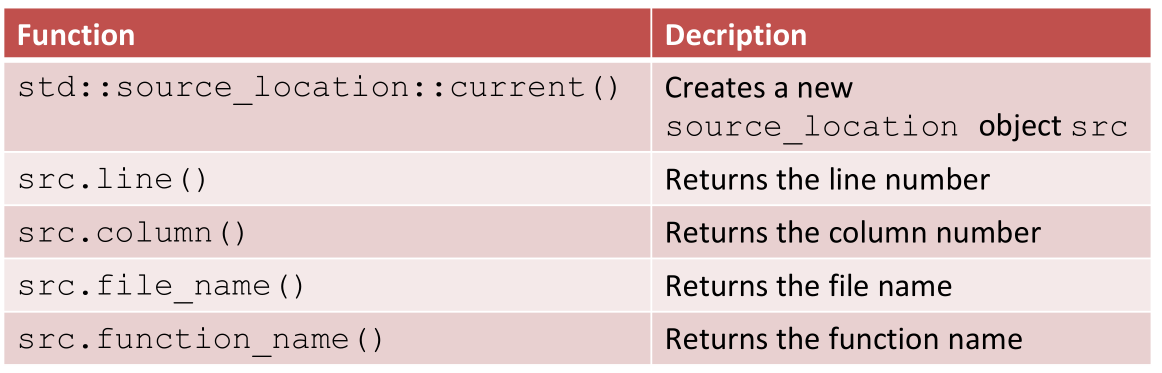The call `std::source_location::current()` creates a new source location object` src. sr`c represents the information of the call site. Now, no C++ compiler supports `std::source_location`. Consequently, the following program `sourceLocation.cpp` is from cppreference.com/source_location.

##Modernes C++ Mentoring

Be part of my mentoring programs:

• "Fundamentals for C++ Professionals" (open)
• "Design Patterns and Architectural Patterns with C++" (open)
• "C++20: Get the Details" (reopens December 2023)
• Do you want to stay informed about my mentoring programs: Subscribe via E-Mail.

```// sourceLocation.cpp
// from cppreference.com

#include <iostream>
#include <string_view>
#include <source_location>

void log(std::string_view message,
const std::source_location& location = std::source_location::current())
{
std::cout << "info:"
<< location.file_name() << ':'
<< location.line() << ' '
<< message << '\n';
}

int main()
{
log("Hello world!");  // info:main.cpp:19 Hello world!
}
```

The output of the program is part of its source code.

C++20 makes it quite comfortable to access or manipulate bits or bit sequences.

## Bit Manipulation

Thanks to the new type std::endian, you get the endianness of a scalar type.

### Endianness

• Endianness can be big-endian or little-endian. Big-endian means the most significant byte comes first; little-endian means that the least significant byte comes first.
• A scalar type is either an arithmetic type, an `enum`, a pointer, a member pointer, or a `std::nullptr_t`.

The class `endian` provides the endianness of all scalar types:

```enum class endian
{
little = /*implementation-defined*/,
big    = /*implementation-defined*/,
native = /*implementation-defined*/
};
```

• If all scalar types are little-endian, `std::endian::native` is equal to `std::endian::little`.
• If all scalar types are big-endian,` std::endian::native` is equal `to std::endian::big`.

Even corner cases are supported:

• If all scalar types have `sizeof` 1 and therefore endianness does not matter; the values of the enumerators `std::endian::little`, `std::endian::big`, and `std::endian::native` are identical.
• If the platform uses mixed endianness, `std::endian::native` is neither equal to `std::endian::big` nor `std::endian::little`.

When I perform the following program `getEndianness.cpp` on an x86 architecture, I get the answer little-endian.

```// getEndianness.cpp

#include <bit>
#include <iostream>

int main() {

if constexpr (std::endian::native == std::endian::big) {
std::cout << "big-endian" << '\n';
}
else if constexpr (std::endian::native == std::endian::little) {
std::cout << "little-endian"  << '\n';      // little-endian
}

}
```

`constexpr if` enables it to compile source code conditionally. This means that the compilation depends on the endianness of your architecture. If you want to know more about endianness, read the same-named Wikipedia page.

### Accessing or Manipulating Bits or Bit Sequences

The following table gives you the first overview of all functions.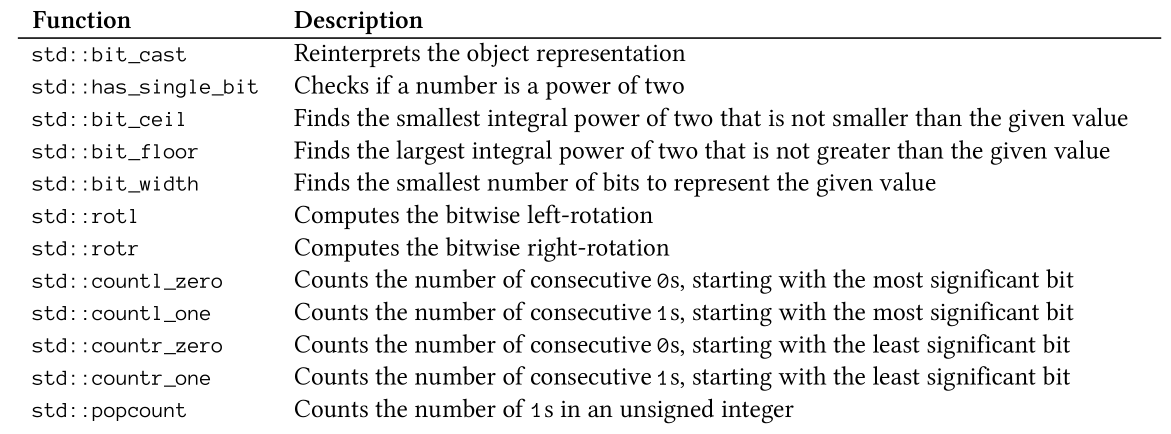The functions except `std::bit_cast` require an unsigned integer type (`unsigned char, unsigned short, unsigned int, unsigned long,` or` unsigned long long`).

The program` bit.cpp` shows the usage of the functions.

```// bit.cpp

#include <bit>
#include <bitset>
#include <iostream>

int main() {

std::uint8_t num= 0b00110010;

std::cout << std::boolalpha;

std::cout << "std::has_single_bit(0b00110010): " << std::has_single_bit(num)
<< '\n';

std::cout << "std::bit_ceil(0b00110010): " << std::bitset<8>(std::bit_ceil(num))
<< '\n';
std::cout << "std::bit_floor(0b00110010): "
<< std::bitset<8>(std::bit_floor(num)) << '\n';

std::cout << "std::bit_width(5u): " << std::bit_width(5u) << '\n';

std::cout << "std::rotl(0b00110010, 2): " << std::bitset<8>(std::rotl(num, 2))
<< '\n';
std::cout << "std::rotr(0b00110010, 2): " << std::bitset<8>(std::rotr(num, 2))
<< '\n';

std::cout << "std::countl_zero(0b00110010): " << std::countl_zero(num) << '\n';
std::cout << "std::countl_one(0b00110010): " << std::countl_one(num) << '\n';
std::cout << "std::countr_zero(0b00110010): " << std::countr_zero(num) << '\n';
std::cout << "std::countr_one(0b00110010): " << std::countr_one(num) << '\n';
std::cout << "std::popcount(0b00110010): " << std::popcount(num) << '\n';

}
```

Here is the output of the program: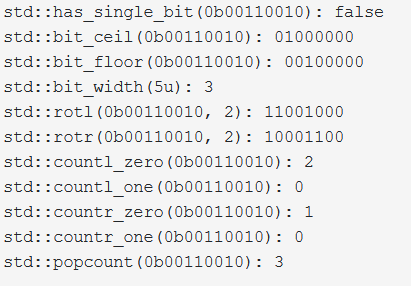The next program shows the application and the output of the functions `std::bit_floor`,` std::bit_ceil`, `std::bit_width`, and `std::bit_popcount` for the numbers 2 to 7.

```// bitFloorCeil.cpp

#include <bit>
#include <bitset>
#include <iostream>

int main() {

std::cout << std::endl;

std::cout << std::boolalpha;

for (auto i = 2u; i < 8u; ++i) {
std::cout << "bit_floor(" << std::bitset<8>(i) << ") = "
<< std::bit_floor(i) << '\n';

std::cout << "bit_ceil(" << std::bitset<8>(i) << ") = "
<< std::bit_ceil(i) << '\n';

std::cout << "bit_width(" << std::bitset<8>(i) << ") = "
<< std::bit_width(i) << '\n';

std::cout << "bit_popcount(" << std::bitset<8>(i) << ") = "
<< std::popcount(i) << '\n';

std::cout << std::endl;
}

std::cout << std::endl;

}
```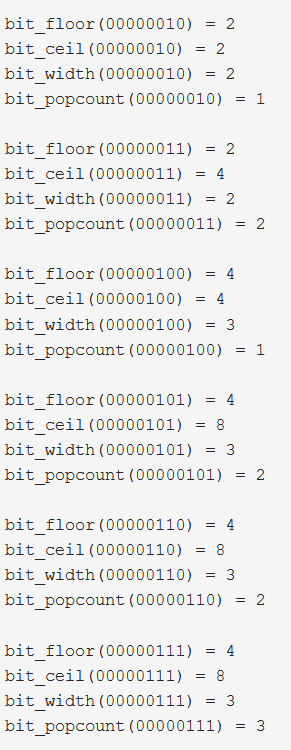## What’s next?

Additionally to coroutines, C++20 has much to offer for concurrency. First, C++20 has new atomics. The new atomics exist for floating-point values and smart pointers. C++20 also enables waiting on atomics. To coordinate threads, semaphores, latches, and barriers come into play. Also, the `std::thread` was improved with `std::jthread`. The execution of a `std::jthread `can be interrupted and joins automatically in its destructor.

Thanks a lot to my Patreon Supporters: Matt Braun, Roman Postanciuc, Tobias Zindl, G Prvulovic, Reinhold Dröge, Abernitzke, Frank Grimm, Sakib, Broeserl, António Pina, Sergey Agafyin, Андрей Бурмистров, Jake, GS, Lawton Shoemake, Jozo Leko, John Breland, Venkat Nandam, Jose Francisco, Douglas Tinkham, Kuchlong Kuchlong, Robert Blanch, Truels Wissneth, Kris Kafka, Mario Luoni, Friedrich Huber, lennonli, Pramod Tikare Muralidhara, Peter Ware, Daniel Hufschläger, Alessandro Pezzato, Bob Perry, Satish Vangipuram, Andi Ireland, Richard Ohnemus, Michael Dunsky, Leo Goodstadt, John Wiederhirn, Yacob Cohen-Arazi, Florian Tischler, Robin Furness, Michael Young, Holger Detering, Bernd Mühlhaus, Matthieu Bolt, Stephen Kelley, Kyle Dean, Tusar Palauri, Dmitry Farberov, Juan Dent, George Liao, Daniel Ceperley, Jon T Hess, Stephen Totten, Wolfgang Fütterer, Matthias Grün, Phillip Diekmann, Ben Atakora, Ann Shatoff, Rob North, Bhavith C Achar, and Marco Parri Empoli.

Thanks, in particular, to Jon Hess, Lakshman, Christian Wittenhorst, Sherhy Pyton, Dendi Suhubdy, Sudhakar Belagurusamy, Richard Sargeant, Rusty Fleming, John Nebel, Mipko, Alicja Kaminska, Slavko Radman, and David Poole.

 My special thanks to EmbarcaderoMy special thanks to PVS-StudioMy special thanks to Tipi.buildMy special thanks to Take Up Code## Seminars

I’m happy to give online seminars or face-to-face seminars worldwide. Please call me if you have any questions.

### Standard Seminars (English/German)

Here is a compilation of my standard seminars. These seminars are only meant to give you a first orientation.

• C++ – The Core Language
• C++ – The Standard Library
• C++ – Compact
• C++11 and C++14
• Concurrency with Modern C++
• Design Pattern and Architectural Pattern with C++
• Embedded Programming with Modern C++
• Generic Programming (Templates) with C++

#### New

• Clean Code with Modern C++
• C++20

### Contact Me

Modernes C++ Mentoring,Tags:
0 replies